### Part 1 - Introduction

This project analyzes NBA Team data from the last 5 seasons (seasons beginning in 2014-2018).

This project aims to answer the questions:

1. Do winning teams have a similar “Three Point Usage Rate” to non-winning teams?

2. Do teams shoot the same number of three pointers in the regular season and playoffs?

### Part 2 - Data

# load packages
library(ggplot2)
library(dplyr)
##
## Attaching package: 'dplyr'
## The following objects are masked from 'package:stats':
##
##     filter, lag
## The following objects are masked from 'package:base':
##
##     intersect, setdiff, setequal, union
library(tidyr)
library(psych)
##
## Attaching package: 'psych'
## The following objects are masked from 'package:ggplot2':
##
##     %+%, alpha
# load data
colnames(nba.data) <- "Team"
colnames(nba.data) <- "Three.Point.Usage.Rate"
colnames(nba.data) <- "Long.Mid.Range.Usage.Rate"
colnames(nba.data) <- "Short.Mid.Range.Usage.Rate"
colnames(nba.data) <- "Paint.Usage.Rate"

library(dplyr)
nba.regseason <- filter(nba.data, SeasonType == "REG")
nba.playoff <- filter(nba.data, SeasonType == "POFF")

The data has 214 cases, each are an NBA Teams’ regular season or post-season.

This study is observational, and the variables of significance are:

1. Response variable: WinPercentage, numerical

Independant variables:

1. ThreePointUsageRate, numberical
2. SeasonType, categorical

### Part 3 - Exploratory data analysis

To explore the data, we will first explore each significant variable individually.

First, exploring Win Percentage

str(nba.data$WinPercentage) ## num [1:214] 0.346 0.598 0.512 0.476 0.268 ... cat("\n") describe(nba.data$WinPercentage)
##    vars   n mean   sd median trimmed  mad min  max range  skew kurtosis
## X1    1 214 0.47 0.18    0.5    0.48 0.16   0 0.94  0.94 -0.43     0.25
##      se
## X1 0.01
cat("\n")
var(nba.data$WinPercentage) ##  0.03243254 cat("\n") summary(nba.data$WinPercentage)
##    Min. 1st Qu.  Median    Mean 3rd Qu.    Max.
##  0.0000  0.3537  0.5000  0.4701  0.5945  0.9375
cat("\n")
cat("IQR of WinPercentage: ", 0.5945-0.3537)
## IQR of WinPercentage:  0.2408
hist(nba.data$WinPercentage, breaks = 20)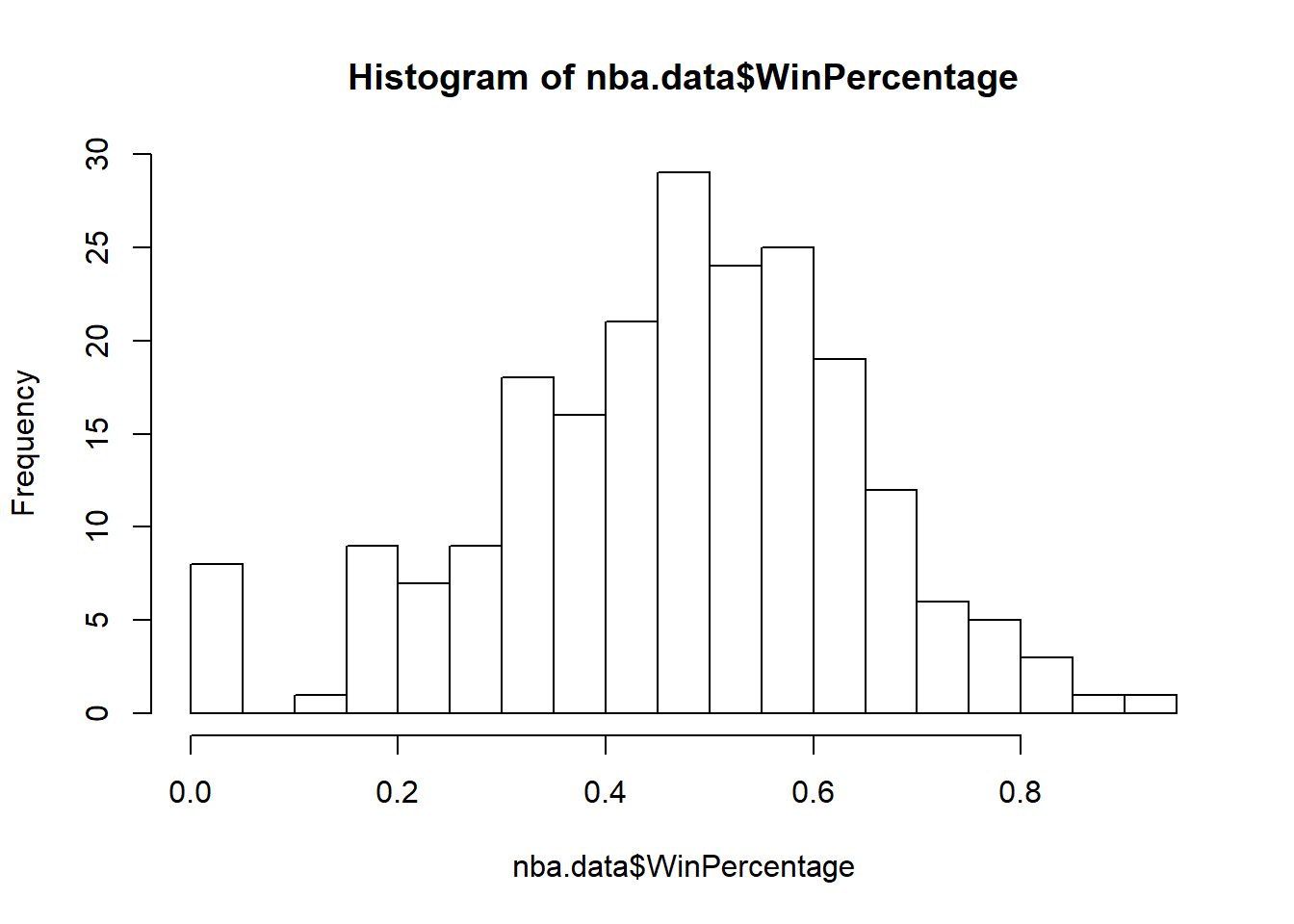qqnorm(nba.data$WinPercentage)
qqline(nba.data$WinPercentage)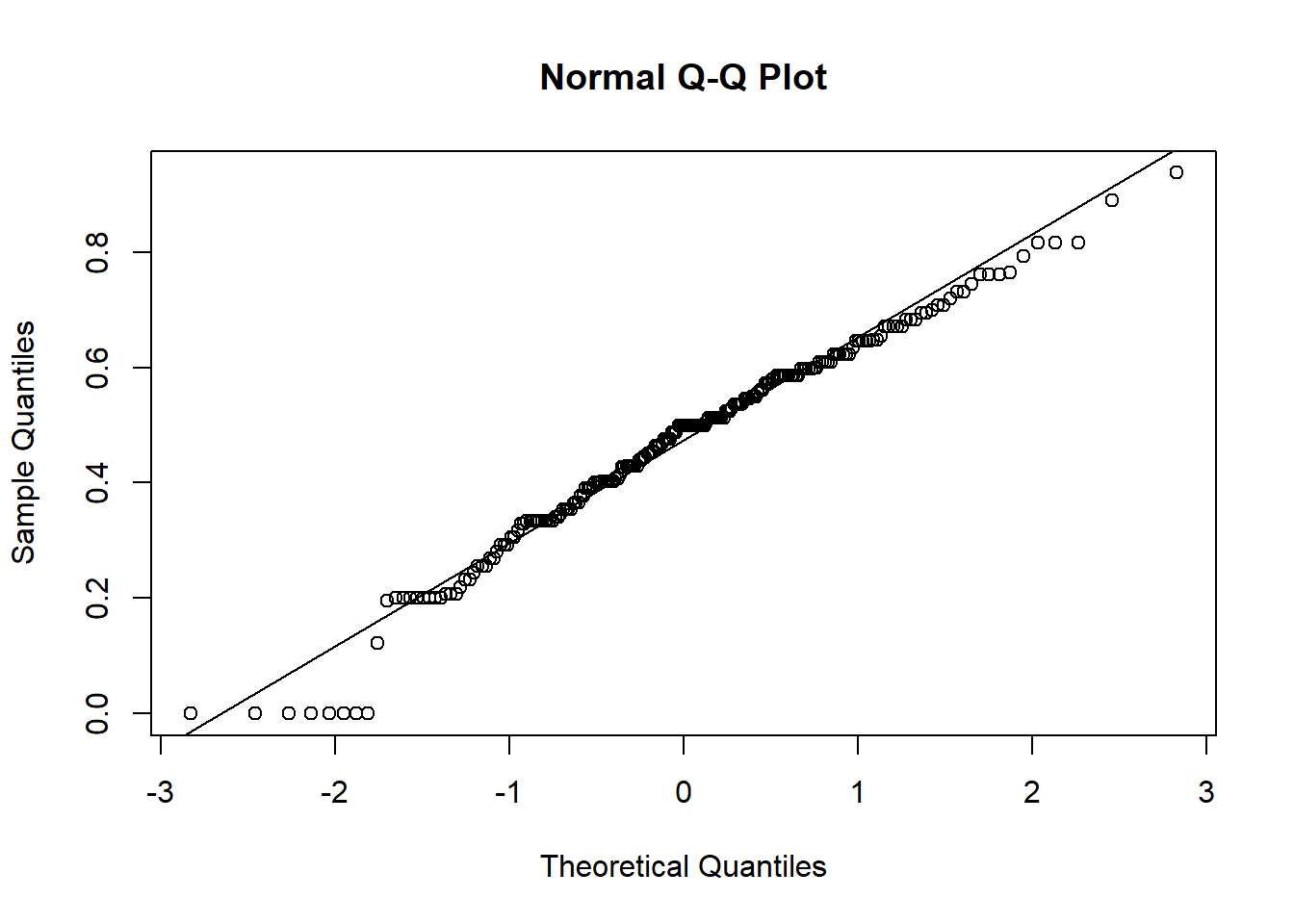boxplot(nba.data$WinPercentage)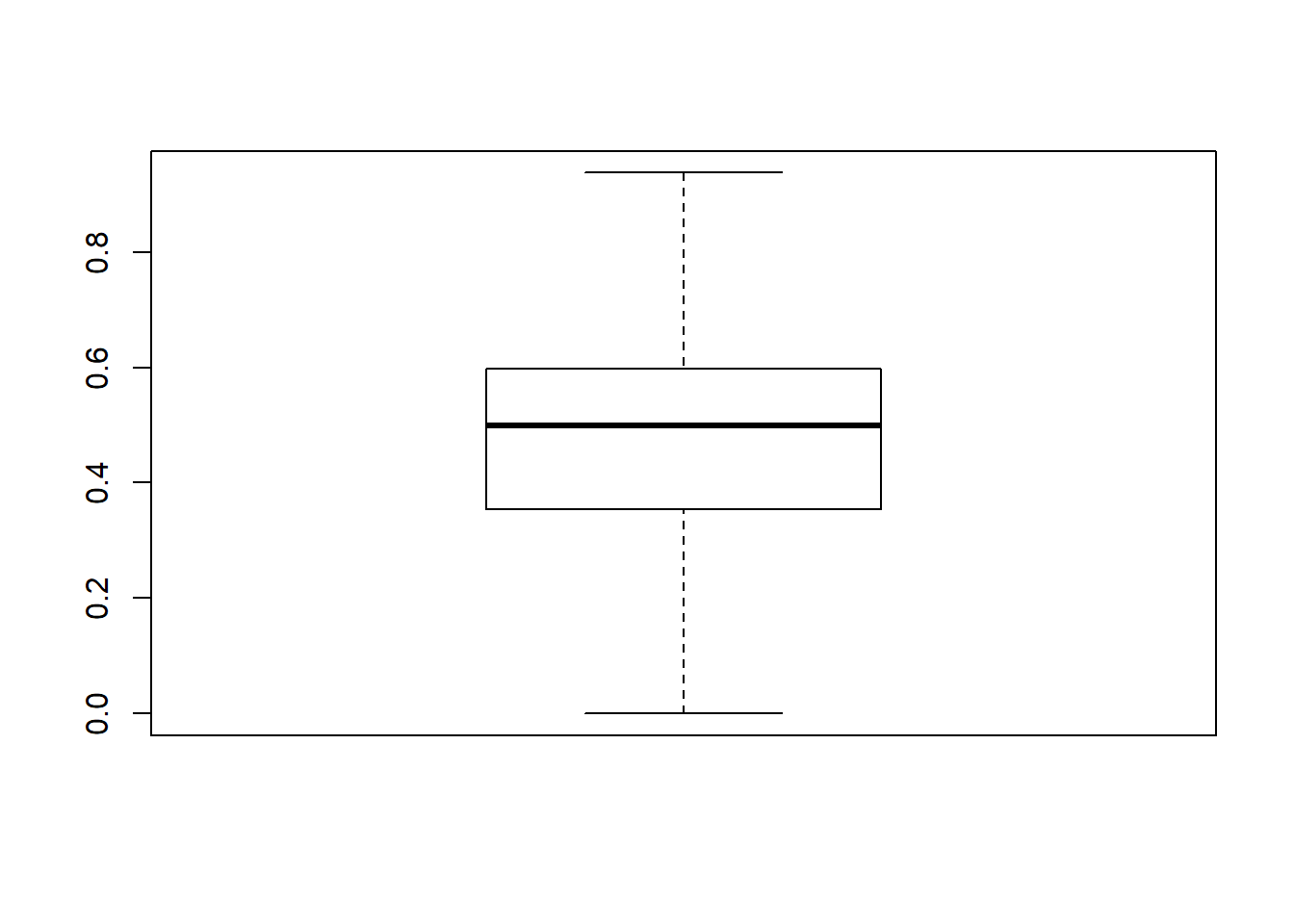Second, to analyze Three.Point.Usage.Rate

str(nba.data$Three.Point.Usage.Rate) ## num [1:214] 39.9 40 38 37.2 29.2 ... cat("\n") describe(nba.data$Three.Point.Usage.Rate)
##    vars   n  mean   sd median trimmed mad   min   max range skew kurtosis
## X1    1 214 31.03 5.78  31.13   30.98   5 16.43 51.74 35.31 0.27     0.72
##     se
## X1 0.4
cat("\n")
var(nba.data$Three.Point.Usage.Rate) ##  33.41813 cat("\n") summary(nba.data$Three.Point.Usage.Rate)
##    Min. 1st Qu.  Median    Mean 3rd Qu.    Max.
##   16.43   27.77   31.13   31.03   34.49   51.74
cat("\n")
cat("IQR of Three.Point.Usage.Rate: ", 34.49-27.77)
## IQR of Three.Point.Usage.Rate:  6.72
hist(nba.data$Three.Point.Usage.Rate, breaks = 20)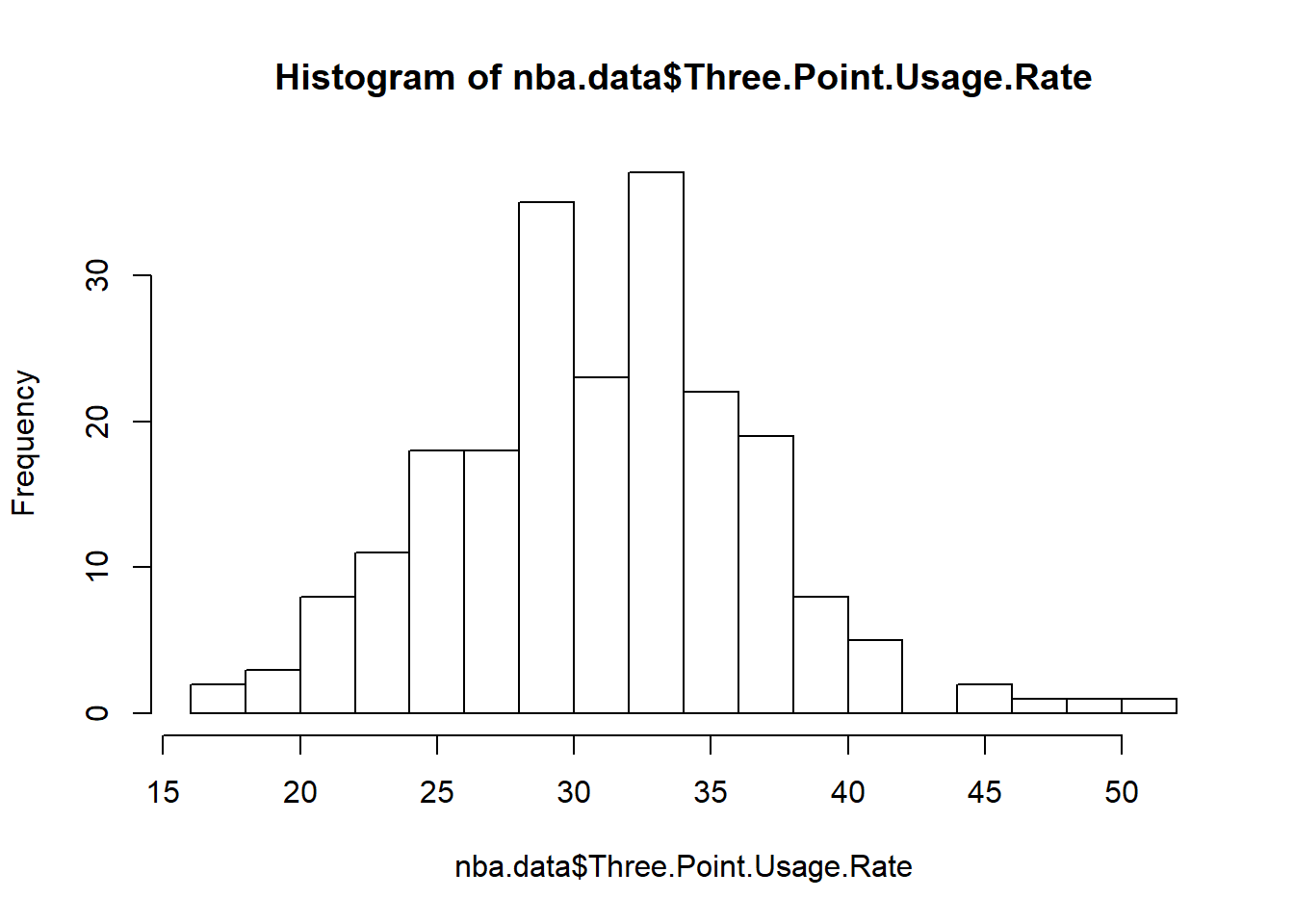qqnorm(nba.data$Three.Point.Usage.Rate)
qqline(nba.data$Three.Point.Usage.Rate)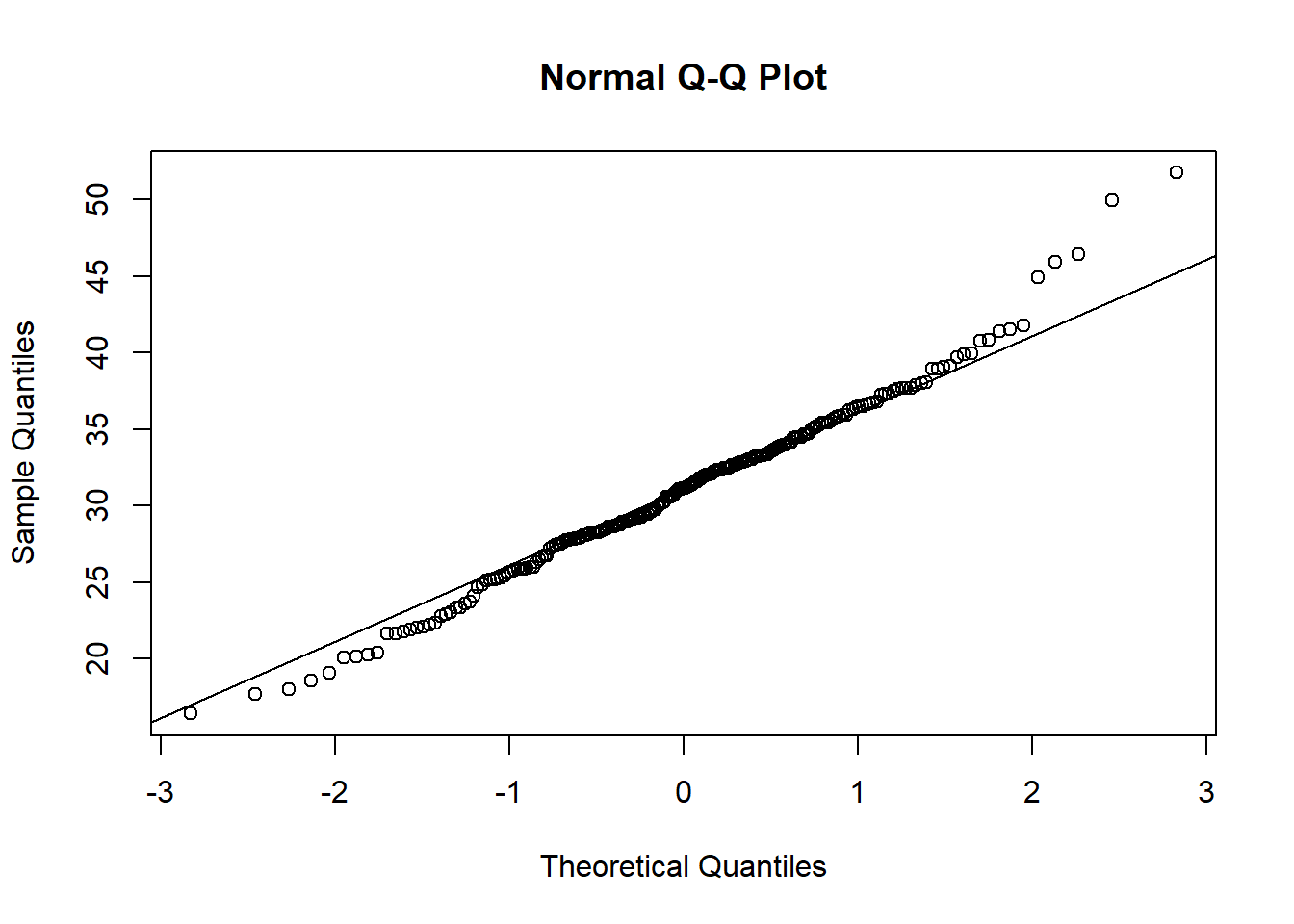boxplot(nba.data$Three.Point.Usage.Rate)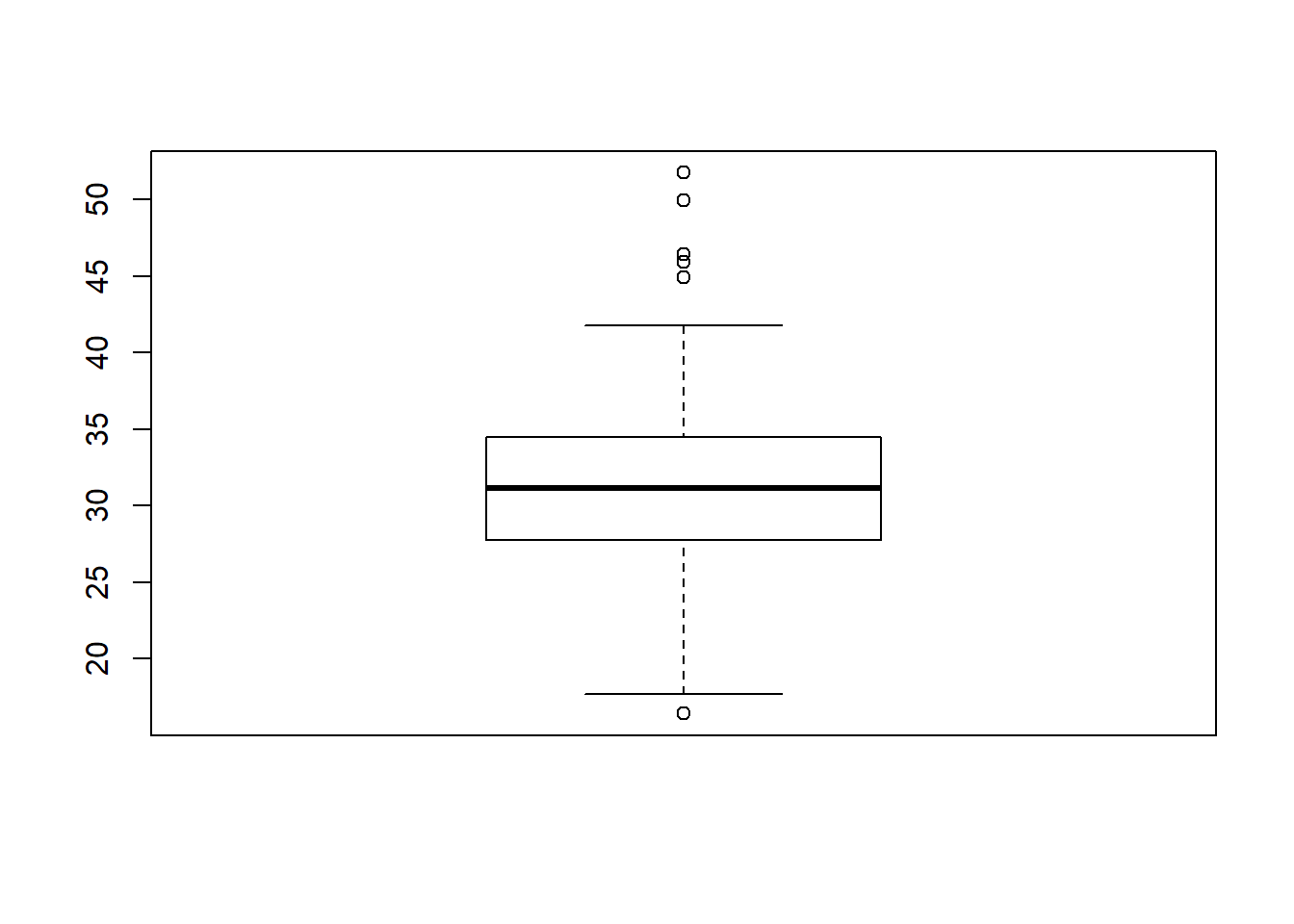# To identify the outliers in the above boxplot for Three.Point.Usage.Rate:

Three.Point.Usage.Outliers_upper <- 34.49 + 1.5*6.72
Three.Point.Usage.Outliers_lower <- 31.13 - 1.5*6.72

three.point.outliers <- subset(nba.data,
Three.Point.Usage.Rate >= Three.Point.Usage.Outliers_upper |
Three.Point.Usage.Rate <= Three.Point.Usage.Outliers_lower)

three.point.outliers %>%
select(Season, SeasonType, Team, Three.Point.Usage.Rate) %>%
arrange(desc(Three.Point.Usage.Rate))
##    Season SeasonType                   Team Three.Point.Usage.Rate
## 1    2018        REG        Houston Rockets                  51.74
## 2    2017        REG        Houston Rockets                  49.94
## 3    2017       POFF        Houston Rockets                  46.41
## 4    2016        REG        Houston Rockets                  45.93
## 5    2016       POFF        Houston Rockets                  44.92
## 6    2014       POFF        Milwaukee Bucks                  20.42
## 7    2014        REG       Sacramento Kings                  20.28
## 8    2014        REG     Washington Wizards                  20.16
## 9    2015        REG Minnesota Timberwolves                  20.06
## 10   2015       POFF      Memphis Grizzlies                  19.08
## 11   2015        REG        Milwaukee Bucks                  18.57
## 12   2014        REG      Memphis Grizzlies                  18.02
## 13   2014        REG Minnesota Timberwolves                  17.73
## 14   2014       POFF      Memphis Grizzlies                  16.43

Lastly, let’s look at SeasonType

summary(nba.data$SeasonType) ## POFF REG ## 64 150 boxplot(nba.data$WinPercentage ~ nba.data$SeasonType)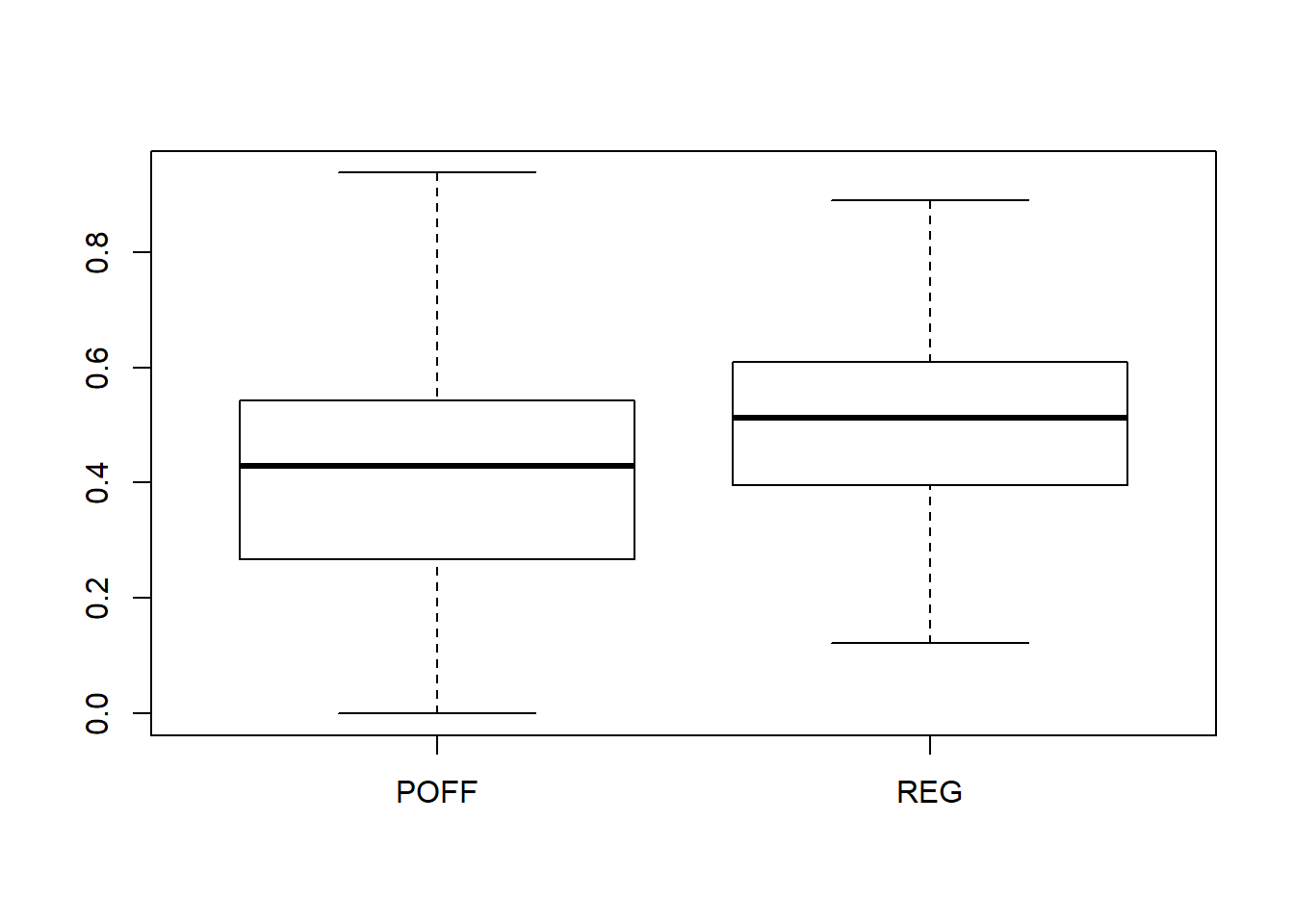boxplot(nba.data$Three.Point.Usage.Rate ~ nba.data$SeasonType)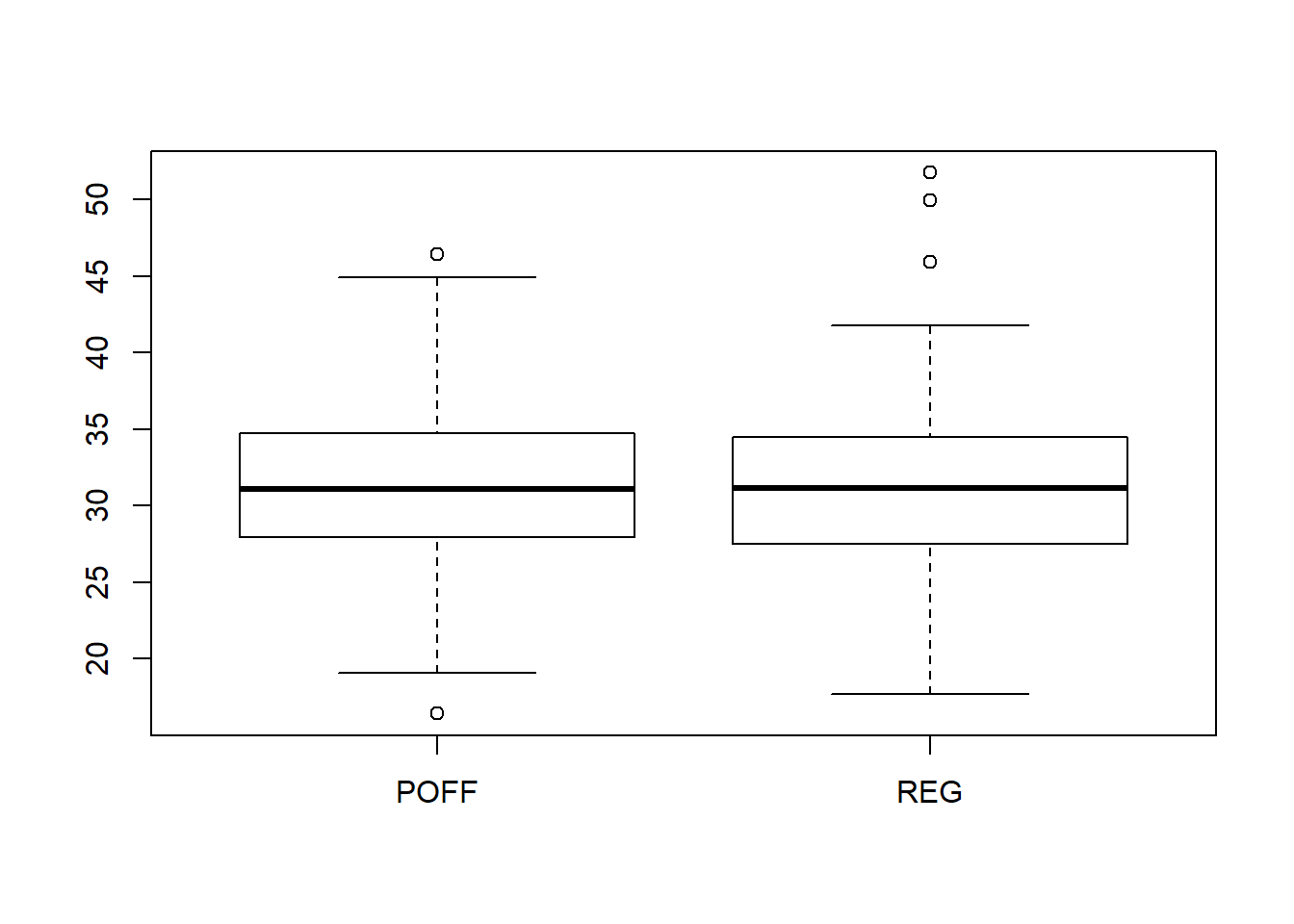mosaicplot(table(nba.data$SeasonType, nba.data$WinPercentage > .5))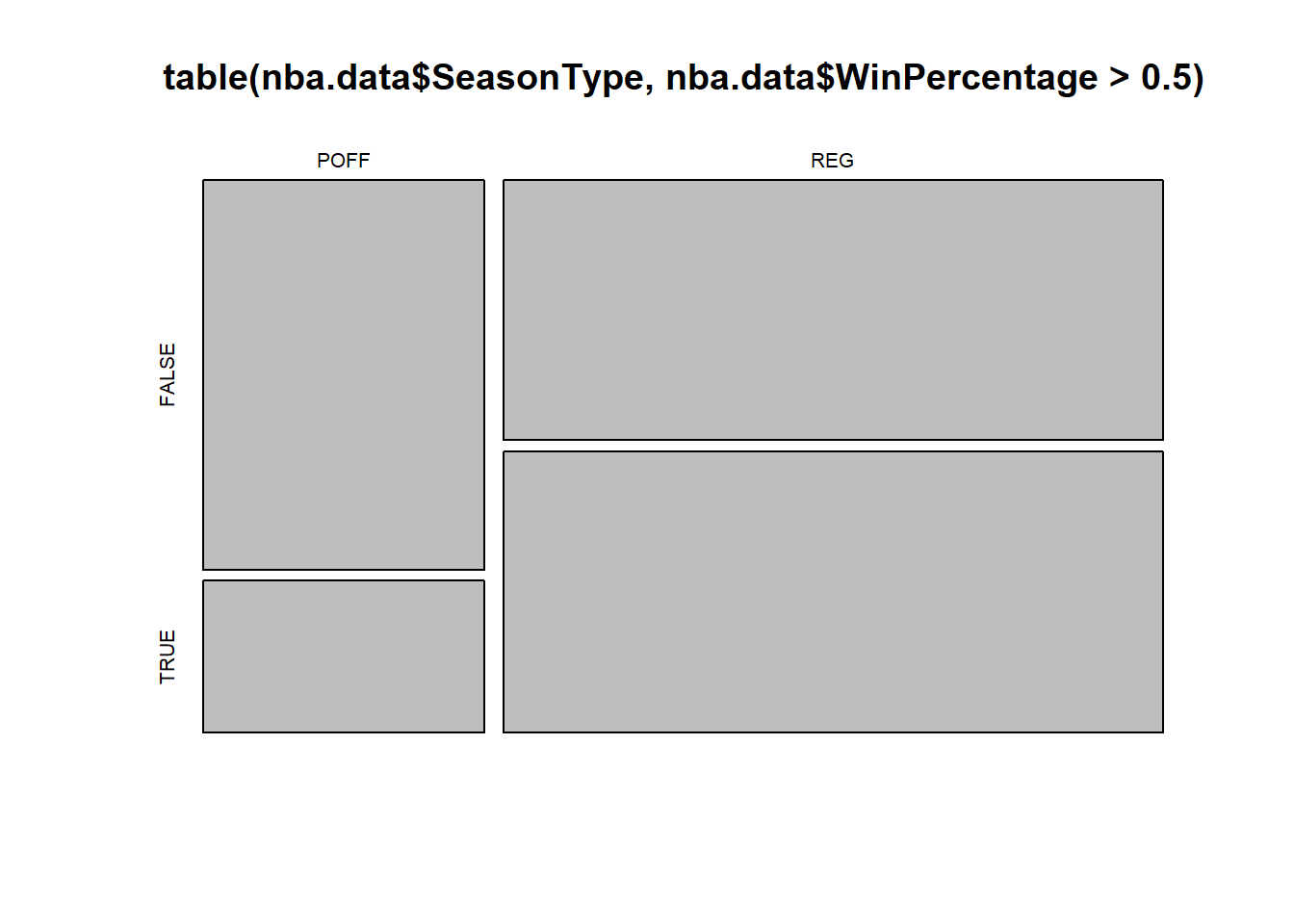ggplot(nba.data, aes(x=Three.Point.Usage.Rate, y=WinPercentage)) + geom_point(shape=23) + geom_smooth() ## geom_smooth() using method = 'loess' and formula 'y ~ x'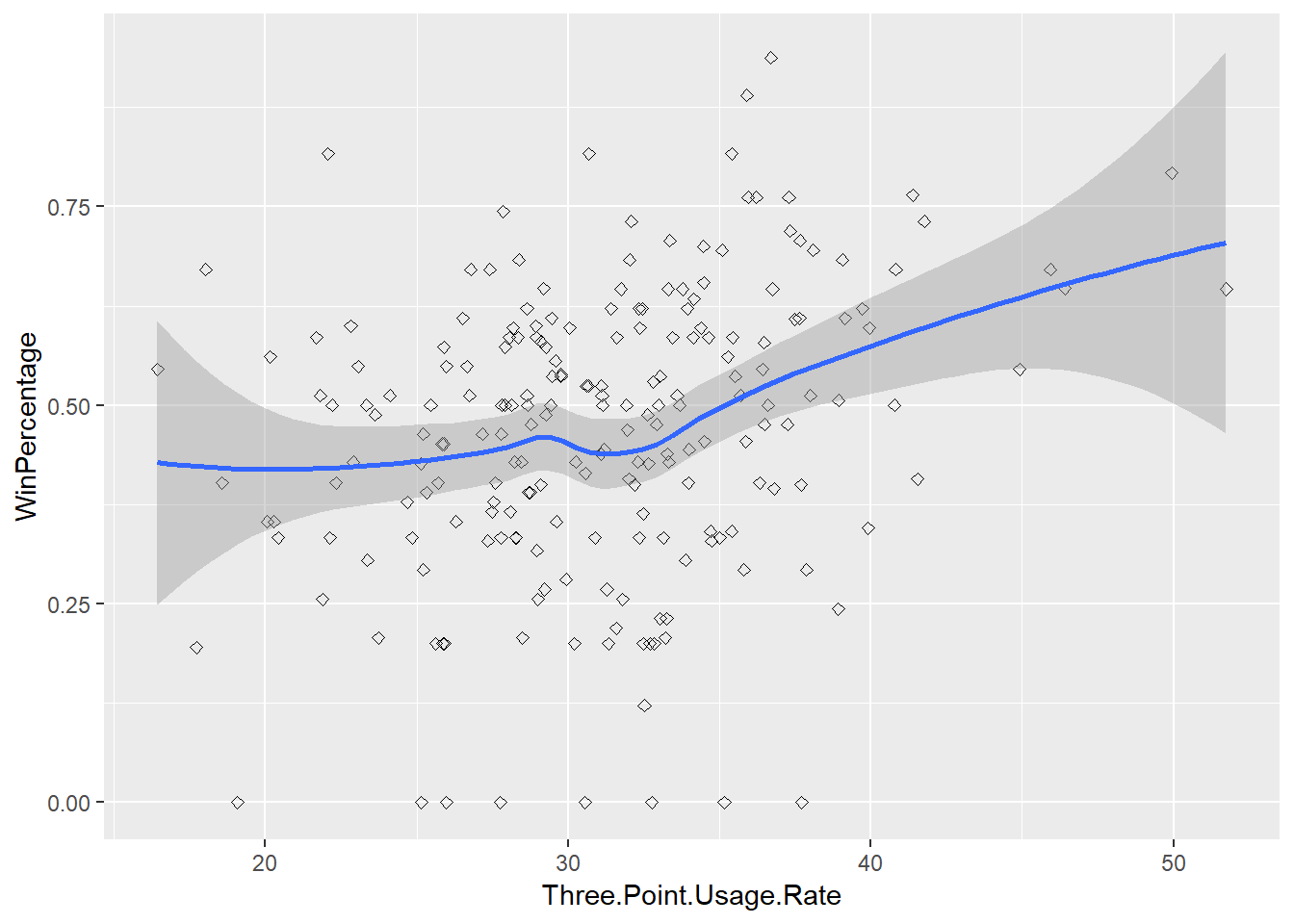There’s a lot to take in from the above exploratory analysis: 1. WinPercentage has a fairly normal distribution, mean = .47, median = .5 2. Three.Point.Usage.Rate has 14 outliers, which are identified above 3. SeasonType creates a significant different in WinPercentage, not as much in Three.Point.Usage.Rate ### Part 4 - Inference Originally, I was going to create a sampling distribution to use for inference. But, I have the entire population of teams for the five year period I am interested in, Seasons 2014-2018. Hence, I will use inference to create the following confidence intervals and test hypothesis’: 1. Confidence Interval for difference between Three.Point.Usage.Rate between Playoffs and Regular Season library(statsr) ## Loading required package: BayesFactor ## Loading required package: coda ## Loading required package: Matrix ## ## Attaching package: 'Matrix' ## The following object is masked from 'package:tidyr': ## ## expand ## ************ ## Welcome to BayesFactor 0.9.12-4.2. If you have questions, please contact Richard Morey (richarddmorey@gmail.com). ## ## Type BFManual() to open the manual. ## ************ inference(y = nba.data$Three.Point.Usage.Rate, x = nba.data$SeasonType, data = nba.data, type = "ci", statistic = "mean", method = "theoretical", order = c("REG", "POFF")) ## Response variable: numerical, Explanatory variable: categorical (2 levels) ## n_REG = 150, y_bar_REG = 31.002, s_REG = 5.8142 ## n_POFF = 64, y_bar_POFF = 31.088, s_POFF = 5.747 ## 95% CI (REG - POFF): (-1.8067 , 1.6347)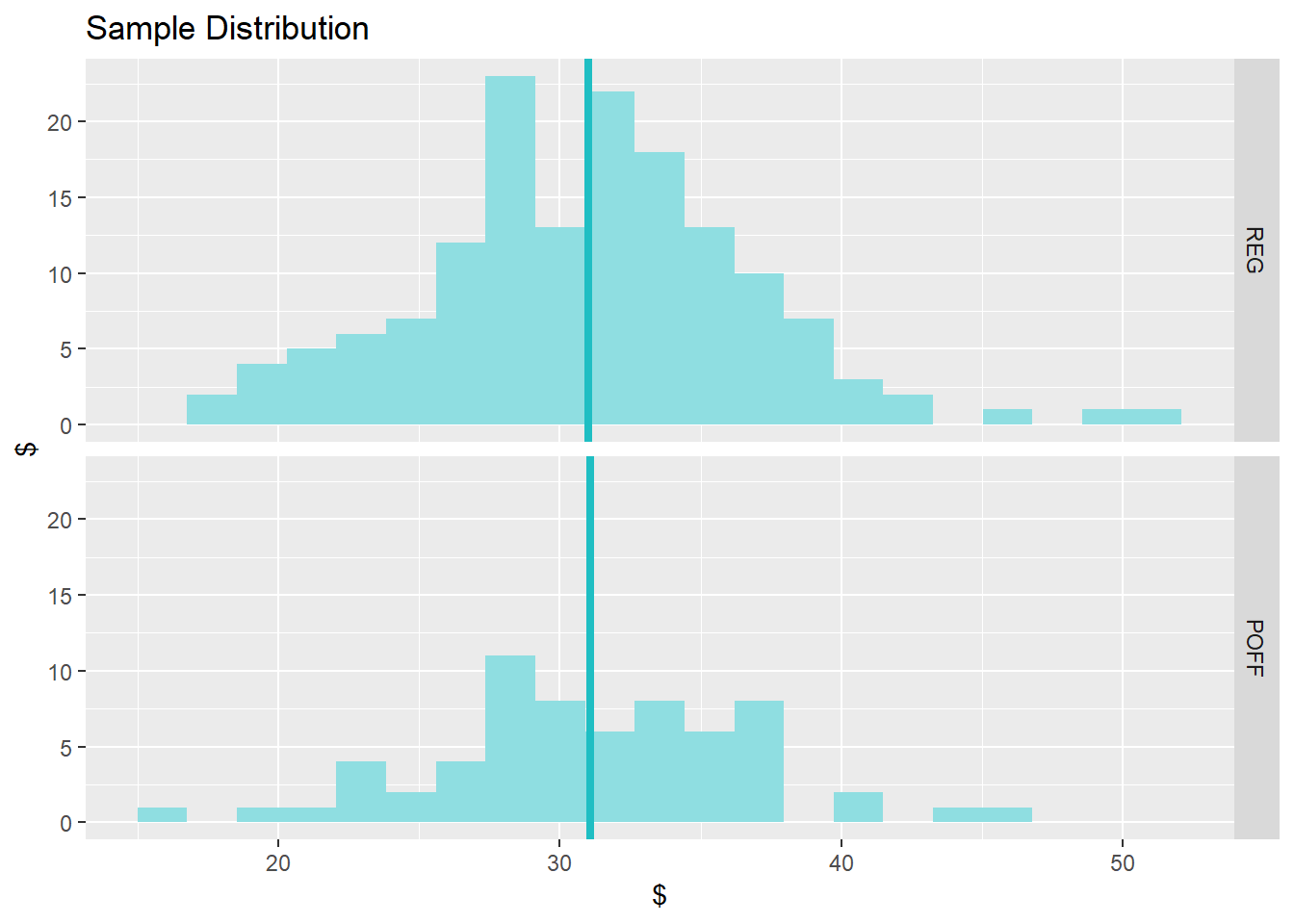1. Hypthesis Test: Do winning teams shoot the same amount of three pointers as non-winning teams in the regular season? Null: Winning teams shoot the same amount of Three Pointers as non-winning teams in the regular season Alternate: Winning teams shoot do not shoot the same amount of Three Pointers as non-winning teams in the regular season nba.data$WinningTeam <- FALSE
for (i in 1:nrow(nba.data)){
if (nba.data$WinPercentage[[i]] > .5){ nba.data$WinningTeam[[i]] <- TRUE
}
}

nba.winning.teams <- subset(nba.data, WinPercentage > .5)

inference(y=nba.data$Three.Point.Usage.Rate, x=nba.data$WinningTeam,
data = nba.data, statistic = "mean", type="ht",
method = "theoretical", alternative = "twosided")
## Warning: Missing null value, set to 0
## Response variable: numerical
## Explanatory variable: categorical (2 levels)
## n_FALSE = 118, y_bar_FALSE = 29.8202, s_FALSE = 4.9315
## n_TRUE = 96, y_bar_TRUE = 32.512, s_TRUE = 6.3982
## H0: mu_FALSE =  mu_TRUE
## HA: mu_FALSE != mu_TRUE
## t = -3.3846, df = 95
## p_value = 0.001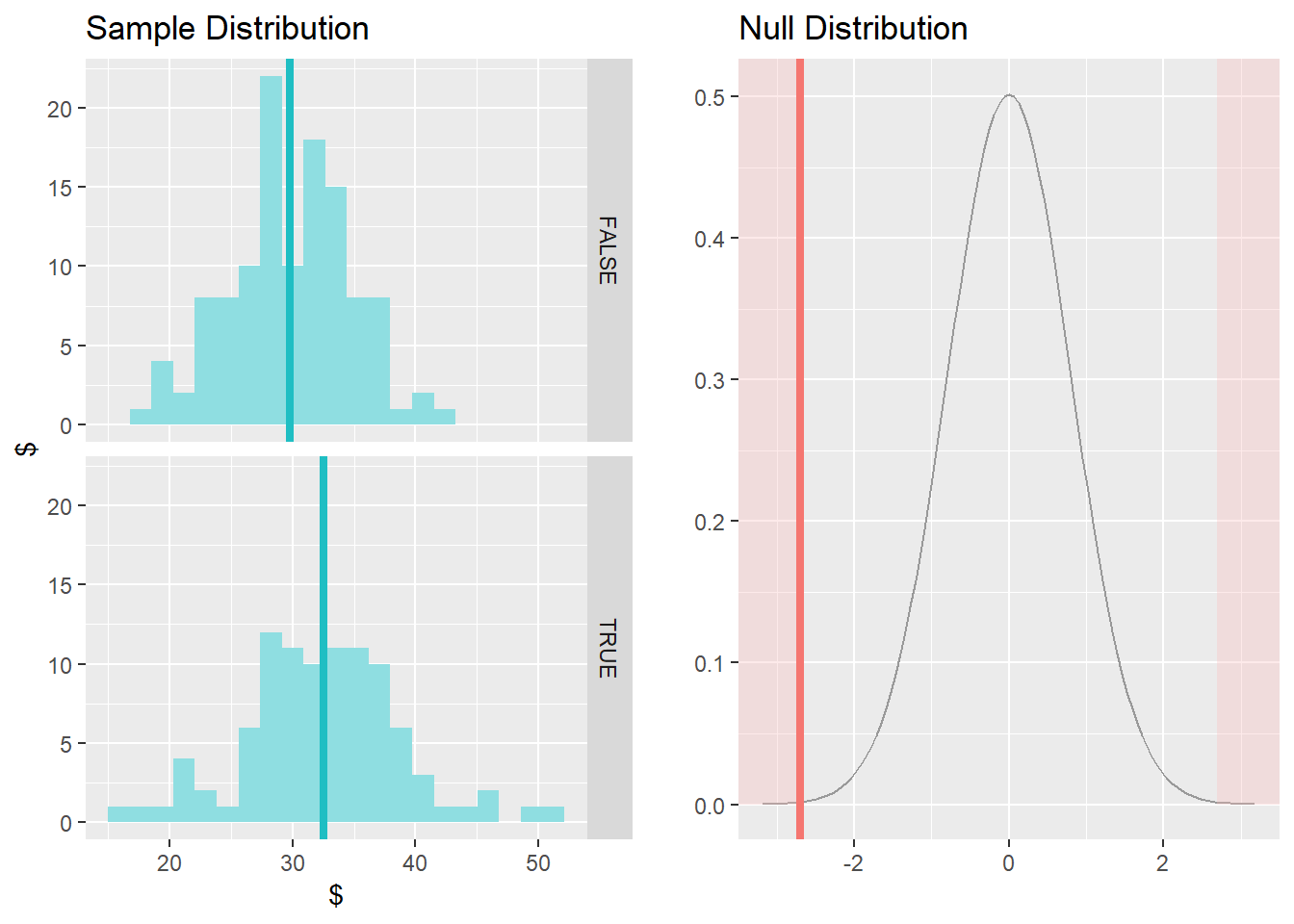1. Hypthesis Test: Do winning teams shoot the same amount of three pointers as non-winning teams in the playoffs?

Null: Winning teams shoot the same amount of Three Pointers as non-winning teams in the playoffs Alternate: Winning teams shoot do not shoot the same amount of Three Pointers as non-winning teams in the playoffs

nba.playoff$WinningTeam <- FALSE for (i in 1:nrow(nba.playoff)){ if (nba.playoff$WinPercentage[[i]] > .5 &
nba.playoff$MatchCount > 4){ nba.playoff$WinningTeam[[i]] <- TRUE
}
}

nba.playoff.winning.teams <- subset(nba.playoff, WinPercentage > .5)

inference(y=nba.playoff$Three.Point.Usage.Rate, x=nba.playoff$WinningTeam,
data = nba.playoff, statistic = "mean", type="ht",
method = "theoretical", alternative = "twosided")
## Response variable: numerical
## Explanatory variable: categorical (2 levels)
## n_FALSE = 46, y_bar_FALSE = 29.9211, s_FALSE = 4.6134
## n_TRUE = 18, y_bar_TRUE = 34.07, s_TRUE = 7.2772
## H0: mu_FALSE =  mu_TRUE
## HA: mu_FALSE != mu_TRUE
## t = -2.2485, df = 17
## p_value = 0.0381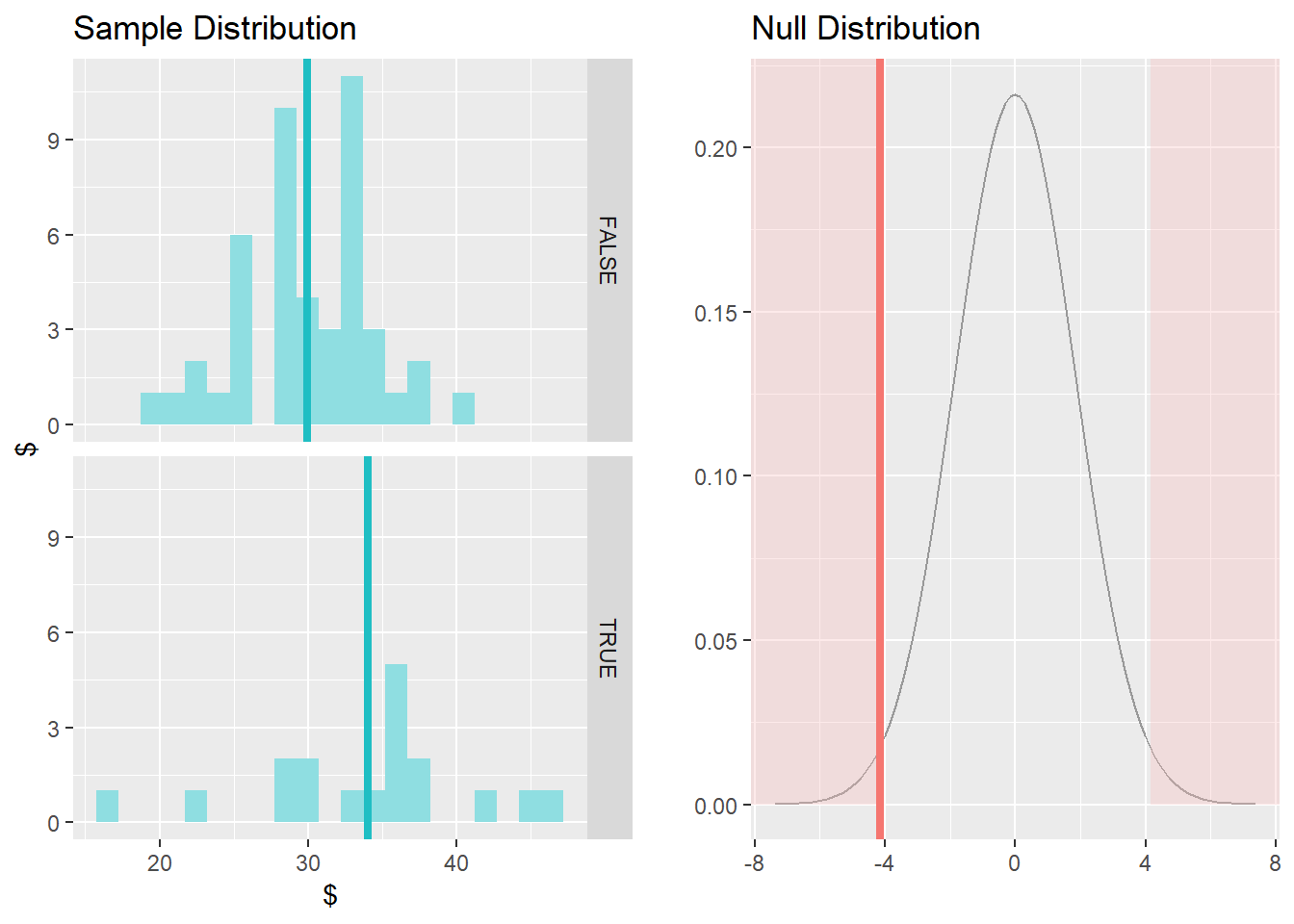### Part 5 - Conclusion

1. Based on Confidence Interval (1) in Part 4, team’s do not shoot more or less three pointers in the playoffs than the regular season

Actually, we can see with 95% confidence that a team will shoot (-1.8067 , 1.6347) more or less threes than the regular season.

1. From Hypothesis Test (2) in Part 4, Winning teams do not shoot the same number of three pointers as non-winning teams in the regular season. (rejected the null hypothesis)

2. From Hypothesis Test (3) in Part 4, Winning teams do not shoot the same number of three pointers as non-winning teams in the playoffs. (rejected the null hypothesis)

3. From the exploratory data analysis, the Houston Rockets are a three point shooting outlier in the last three regular seasons (just as the NBA media suggested).